# Solving LeetCode’s ‘Container with Most Water’ Problem in JavaScript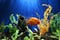Photo by Delbert Pagayona on Unsplash

In this article, we will be solving LeetCode’s ‘Container With Most Water’ problem in JavaScript. This problem uses the two-pointer approach. The two-pointer approach is usually used to keep track of array or string indices to solve a problem. One pointer starts from the beginning while the other pointer starts from the end. The pointers move toward each other until they both meet.

# Problem

Given `n` non-negative integers `a1, a2, ..., an` , where each represents a point at coordinate `(i, ai)`. `n` vertical lines are drawn such that the two endpoints of the line `i` is at `(i, ai)` and…

# Solving LeetCode’s Interval List Intersections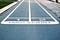Photo by Tim Gouw on Unsplash

In this article, we will be solving LeetCode’s Interval List Intersections in JavaScript. This problem uses the two-pointer approach. The two-pointer approach is usually used to keep track of array or string indices to solve a problem. One variation is one pointer being slow and the other pointer being fast meaning that the fast pointer is ahead of the slow pointer while traversing through the array/string. A classic example of this is to remove duplicates from a sorted array. Another variation is one pointer starts from the beginning while the other pointer starts from the end. The pointers move toward…

# Solving LeetCode’s ‘Maximum Number of Vowels in a Substring of Given Length’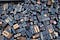Photo by Amador Loureiro on Unsplash

In this article, we will be solving LeetCode’s Maximum Number of Vowels in a Substring of Given Length in JavaScript. This problem uses the sliding window technique. There are two types of sliding window. The technique used for this problem is a fixed window length k. We shift the window over to find something specific in the window such as the maximum number in the window. The other technique involves 2 pointers (usually indices of string/array) representing 2 edges on the side of the window. Start index is on the left edge and end index is on the right edge…

# Asynchronous Fruits with async and await JavaScript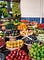Photo by Reiseuhu on Unsplash

`async` and `await` are two keywords that can help make asynchronous code read more like synchronous code. This can help code look cleaner while keeping the benefits of asynchronous code. Asynchronous means that things can happen independently of the main program flow. Most programs are asynchronous and pause their execution until they are needed, allowing the computer to execute other things in the meantime.

`async` and `await` are functions built on promises. A promise is commonly defined as a proxy for a value that will eventually become available. Promises are one way to deal with asynchronous code.

# How do promises work?

A `Promise` is…

# Solving LeetCode’s ‘Swapping Nodes in a Linked List’

In this article, we will be solving LeetCode’s Swapping Nodes in a Linked List in JavaScript.

Here is an article I wrote on Linked Lists and goes through a LeetCode problem.

Recap, a linked list is a linear collection of nodes where each node points to the next node. Each node has a value property and a next property which references the next node in the list.

This is what makes linked lists a linear data structure. There is a sequence and an order to the list. To get to the end of the list, we must start at the…

# How to Solve the ‘Find Center of Star Graph’ in JavaScript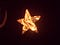Photo by Michael Kilcoyne on Unsplash

In this article, we will be solving LeetCode’s Find Center of Star Graph in JavaScript. This article will be using what we have learned from my “Intro to Graphs” series.

Part 1 was the general introduction of what graphs are. In summary, a graph data structure is a finite set of vertices (nodes) and a set of edges that connect a pair of nodes. In graphs, there are no rules dictating the connection among the nodes. The edges can connect nodes no matter their placement.

Part 2 was learning about the two standard approaches of representing graphs, an adjacency matrix…

# Intro to Graphs (part 3)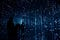Photo by Robynne Hu on Unsplash

Hello all! This is part 3 of the series Intro to Graphs. Part 1 was the general introduction of what graphs are. In summary, a graph data structure is a finite set of vertices (nodes) and set of edges which connect a pair of nodes. In graphs, there are no rules dictating the connection among the nodes. The edges can connect nodes no matter their placement. Part 2 was learning about the two standard approaches of representing graphs, an adjacency matrix and an adjacency list. This article will be going over graph traversal.

# Practical uses

Why do we need to know graph…

# Intro to Graphs (part 2)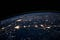Photo by NASA on Unsplash

Hello all! This is part 2 of the series Intro to Graphs. If you missed part 1, the link is here and at the bottom of this article. Recap, a graph data structure is a finite set of vertices (nodes) and set of edges which connect a pair of nodes. In graphs, there are no rules dictating the connection among the nodes. The edges can connect nodes no matter their placement. In this article, we will go over representing graphs. The two standard approaches to representing graphs are an adjacency matrix and an adjacency list.

# Intro to Graphs (part 1)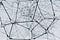Photo by Alina Grubnyak on Unsplash

A graph data structure is a finite set of vertices (nodes) and set of edges which connect a pair of nodes. In graphs, there are no rules dictating the connection among the nodes. The edges can connect nodes no matter their placement.

# Solving LeetCode’s Last Stone Weight in JavaScript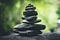Photo by Sean Stratton on Unsplash

Link to the problem is here and in the resources section.

# Problem

We have a collection of stones, each stone has a positive integer weight.

Each turn, we choose the two heaviest stones and smash them together. Suppose the stones have weights x and y with x <= y. The result of this smash is:

• If x == y, both stones are totally destroyed;
• If x != y, the stone of weight x is totally destroyed, and the stone of weight y has new weight y-x.

At the end, there is at most 1 stone left. Return the weight of this…## Chandler Hanson

Flatiron School software engineering alum. Experienced in JavaScript, React.js, Ruby, and Ruby on Rails. https://www.linkedin.com/in/chandler-hanson/